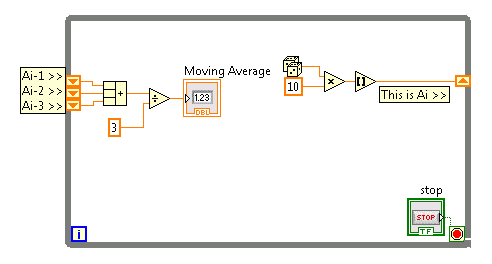# Real-Time Measurement and Control

cancel
Showing results for
Did you mean:

Highlighted

## how to save temporarily the data when real time acquisition

Hello,

I have a problem on data saving when I did real time data acquisiton.

3-second ECG signal each time was acquired to calculate a parameter by matlab script. this parameter should be saved temporarily, then the second 3-second ECG signal was got to calculate this parameter, then it is saved temporarily, then the third calculated parameter will be averaged with the last two parameters, then the average value was output.

for example, the parameters in 15 seconds are: A1,A2,A3,A4,A5. the average value at first is (A1+A2+A3)/3, then the next average value is (A2+A3+4)/3,

then the third one is (A3+A4+A5)/3.

so in order to calculate the average value, I have to save temporarily the parameters. How to save them, which function can be used ?

Thank you

Message 1 of 2
(2,719 Views)
Highlighted

## Re: how to save temporarily the data when real time acquisition

If you're running in a loop, you should be able to use stacked shift registers

Here's an exampleMessage 2 of 2
(2,716 Views)